## 1 GAN

### 1.1 Introduction

To learn the generator’s distribution $p_g$ over data x, we define a prior on input noise variables $p_z(z)$, then represent a mapping to data space as $G(z; θ_g)$, where $G$ is a differentiable function represented by a multilayer perceptron with parameters $θ_g$. We also define a second multilayer perceptron $D(x; θ_d)$ that outputs a single scalar. $D(x)$ represents the probability that $x$ came from the data rather than $p_g$. We train $D$ to maximize the probability of assigning the correct label to both training examples and samples from $G$. We simultaneously train $G$ to minimize $log(1 - D(G(z)))$. In other words, $D$ and $G$ play the following two-player mini-max game with value function $V (G; D):$

$$min_G max_DV (D; G) = E_x∼p_{data(x)}[log D(x)] + E_{z∼p_z(z)}[log(1 - D(G(z)))]$$

### 1.2 Theory Analysis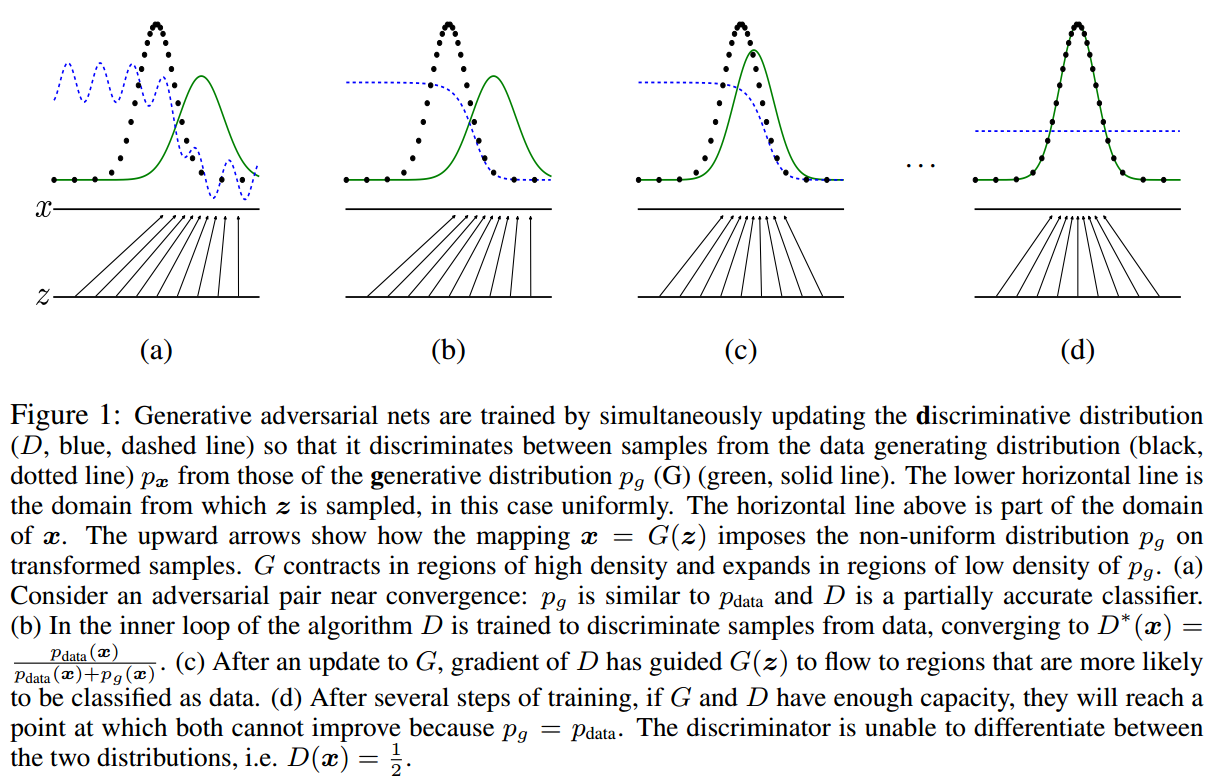## 2.1 Task: Cross Domain Image Translation

Image-to-image translation is a class of vision and graphics problems where the goal is to learn the mapping between an input image and an output image.

In this paper, we present a method that can learn to do the same: capturing special characteristics of one image collection and figuring out how these characteristics could be translated into the other image collection, all in the absence of any paired training examples.

### 2.2 Model: Cycle consistent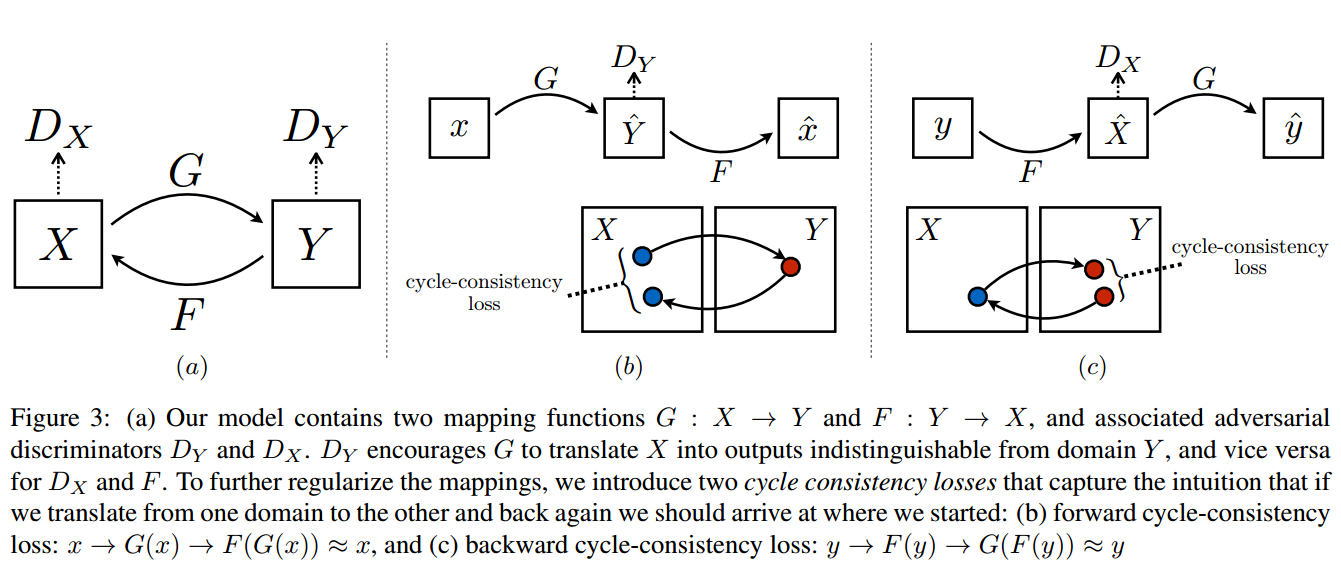Loss: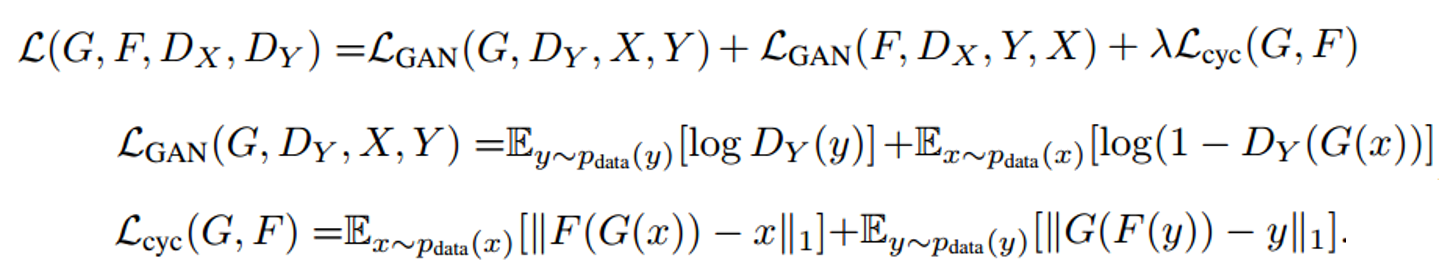### 2.3 Experiment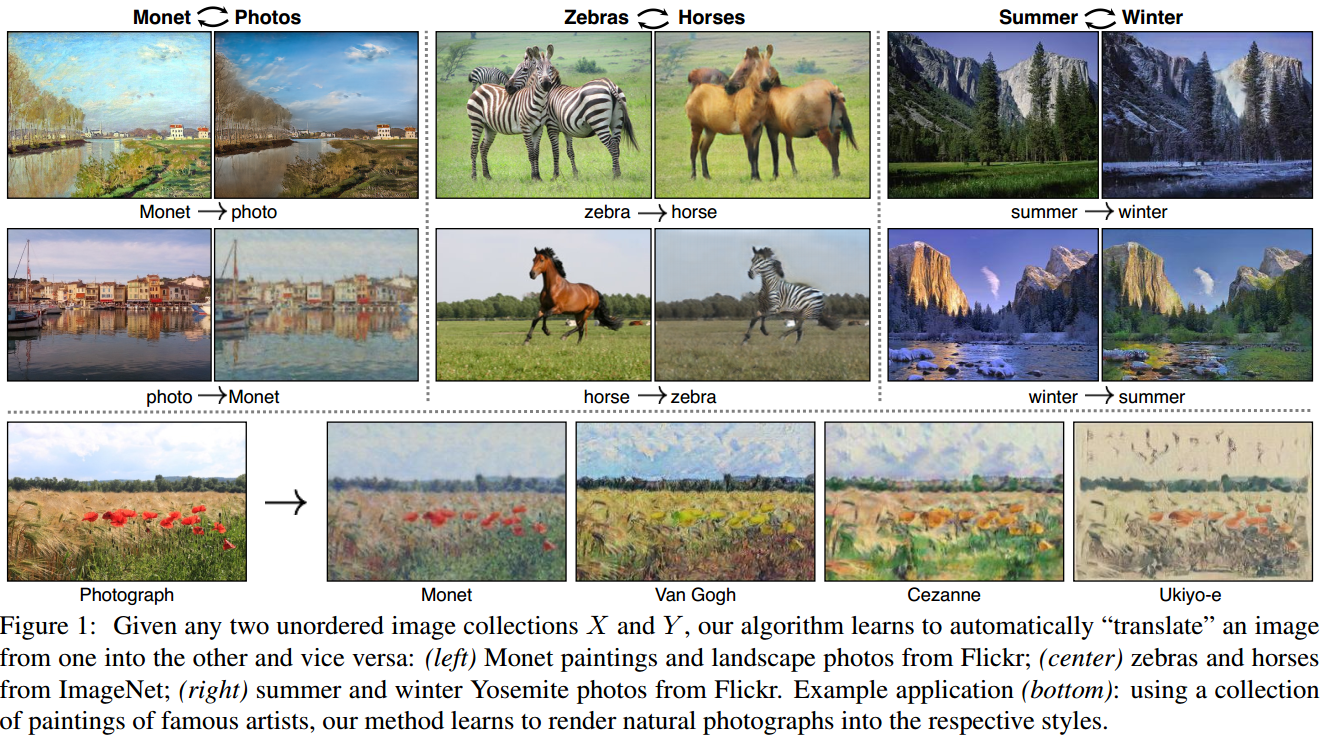1. Dataset: Cityscapes dataset , map and aerial photo on data scraped from Google Maps

2. Metrics: AMT perceptual studies, FCN score, Semantic segmentation metrics

3. Result: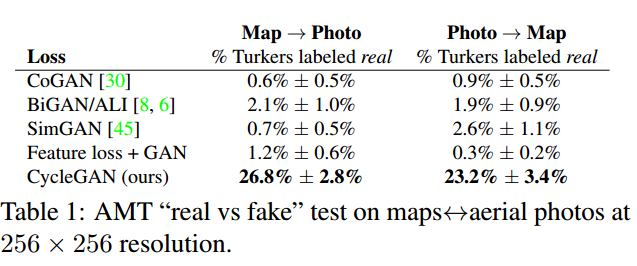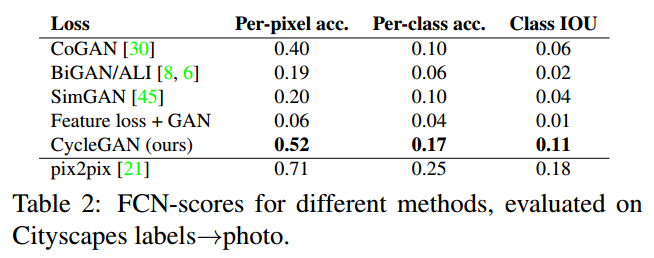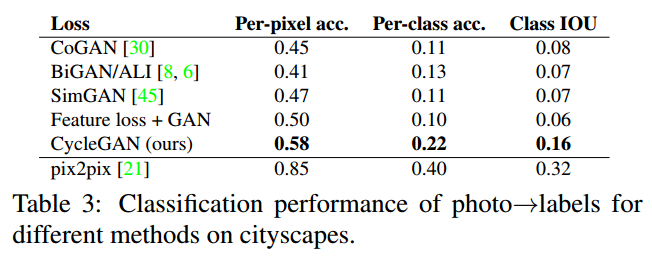### 2.4 Limitations

1. On translation tasks that involve color and texture changes, like many of those reported above, the method often succeeds. We have also explored tasks that require geometric changes, with little success.
2. Some failure cases are caused by the distribution characteristics of the training datasets.
3. We also observe a lingering gap between the results achievable with paired training data and those achieved by our unpaired method.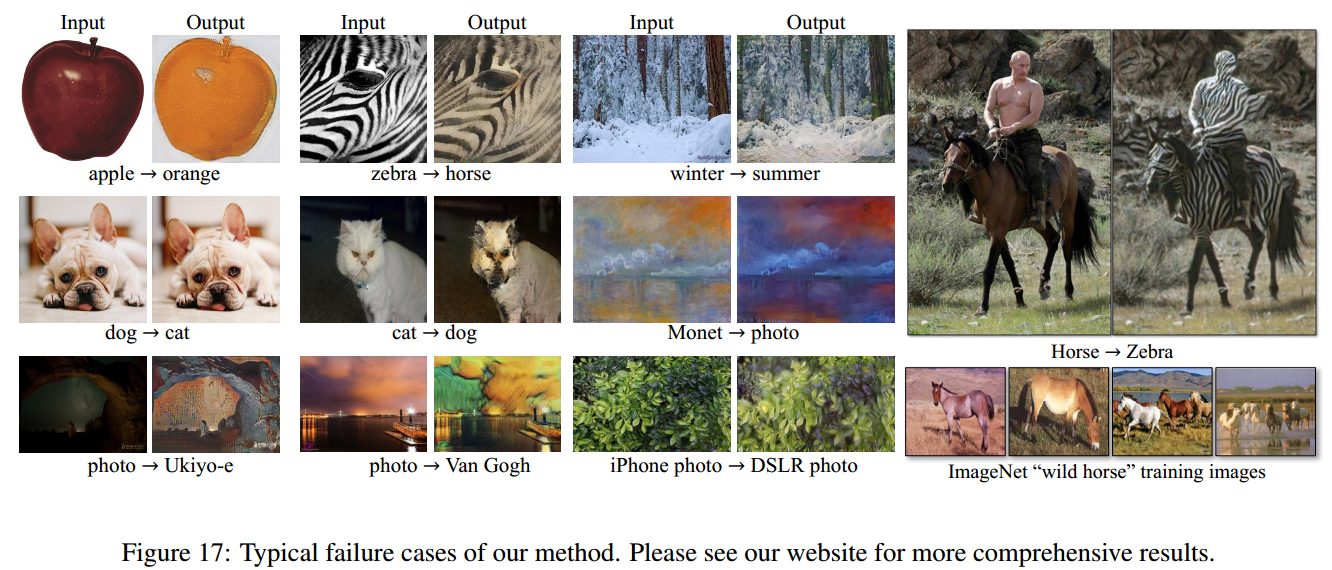## 3 DIAT: Deep Identity-aware Transfer of Facial Attributes

### 3.1 Task: Identity-aware Transfer of Facial Attributes

Our DIAT and DIAT-A models can provide a unified solution for several representative facial attribute transfer tasks such as expression transfer, accessory removal, age progression, and gender transfer

### 3.2 Model

In this section, a two-stage scheme is developed to tackle the identity-aware attribute transfer task.

1. Face transform network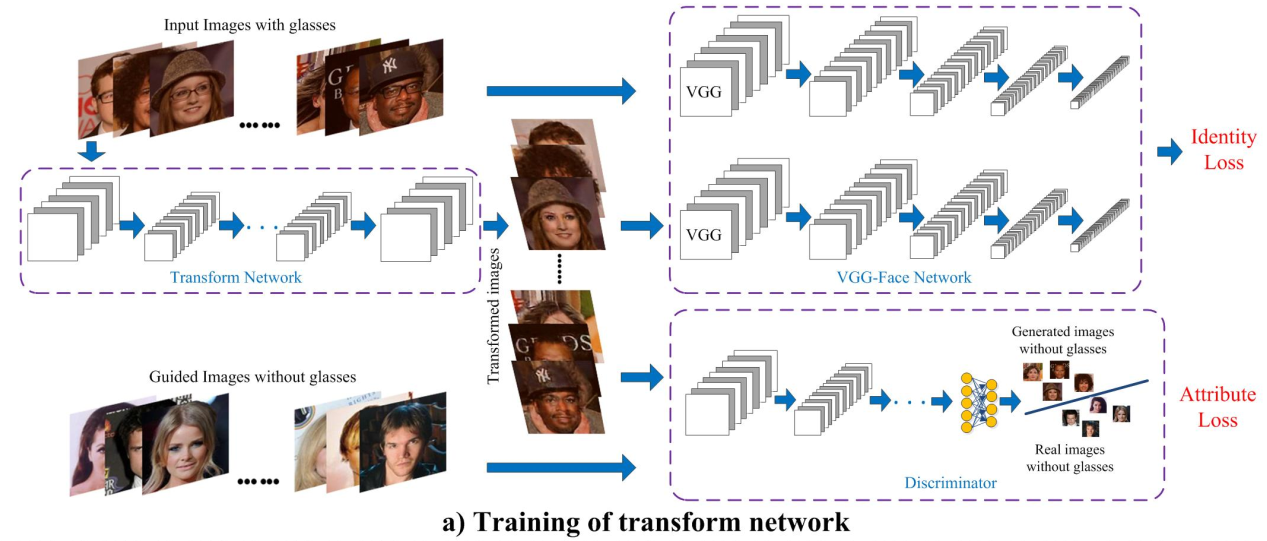Loss: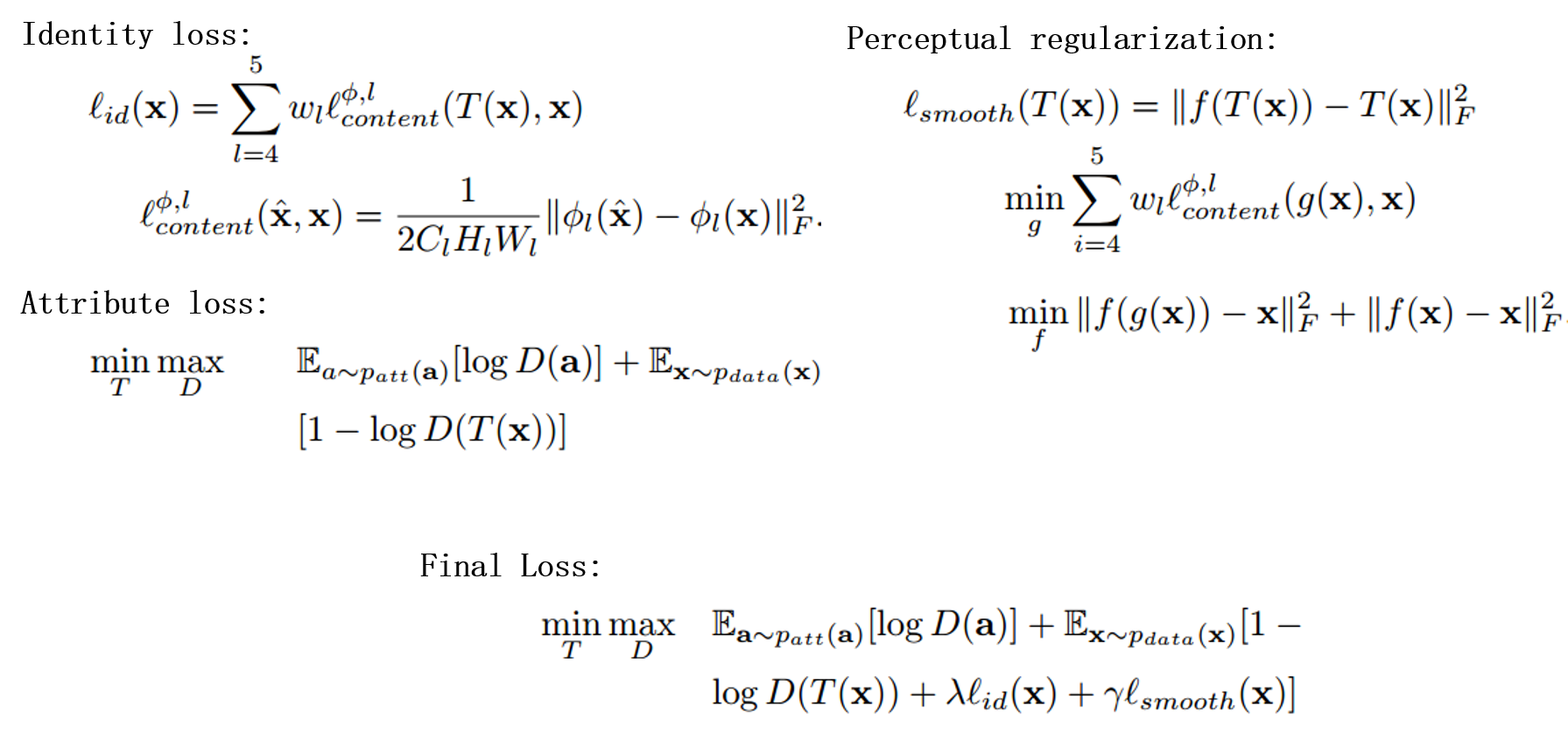2. Face Enhancement Network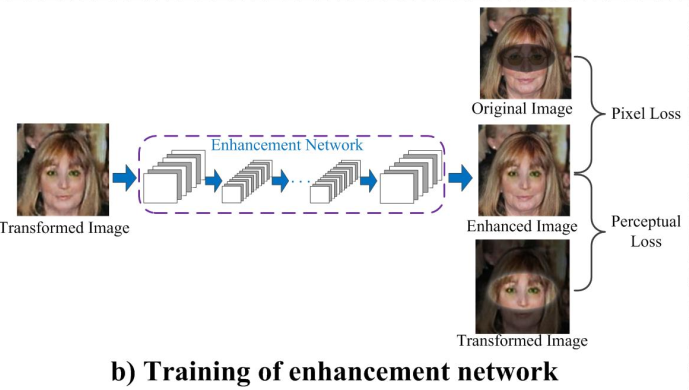Loss: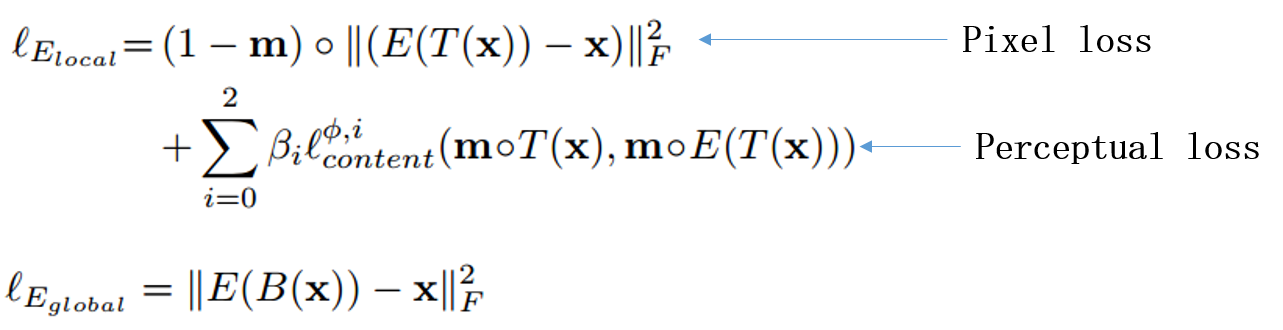### 3.3 DIAT-A

In DIAT, the perceptual identity loss is defined on the pre-trained VGG-Face. Actually, it may be more effective to define this loss on some CNN trained to attribute transfer. Here we treat identity-preserving and attribute transfer as two related tasks, and define the perceptual identity loss based on the convolutional features of the discriminator. By this way, the network parameters for identity loss will be changed along with the updating of discriminator, and thus we named it as adaptive perceptual identity loss.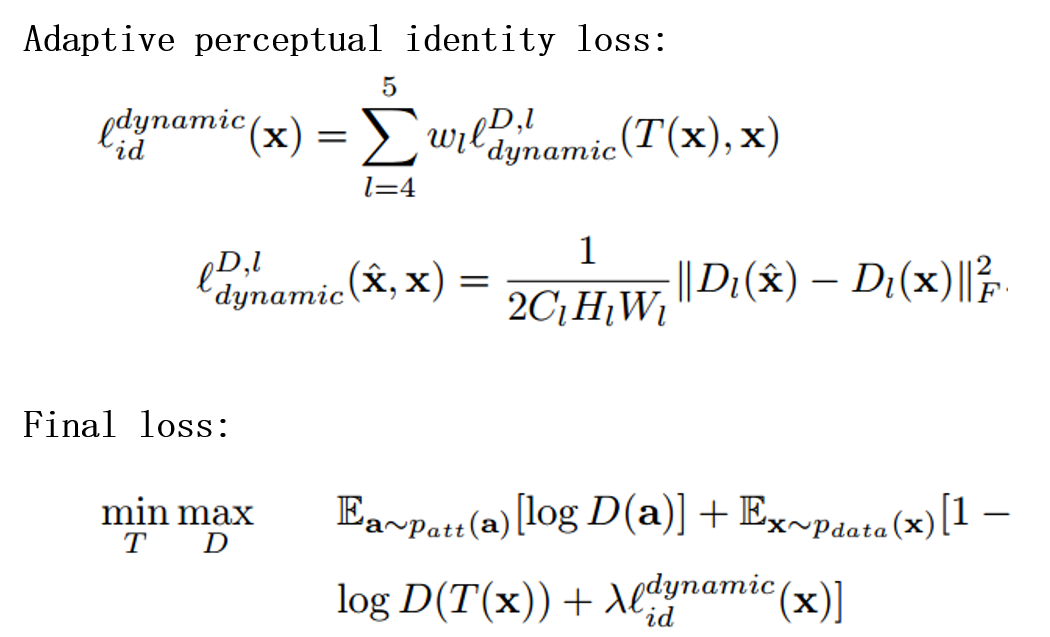### 3.4 Experiments

Dataset: a subset of the aligned CelebA dataset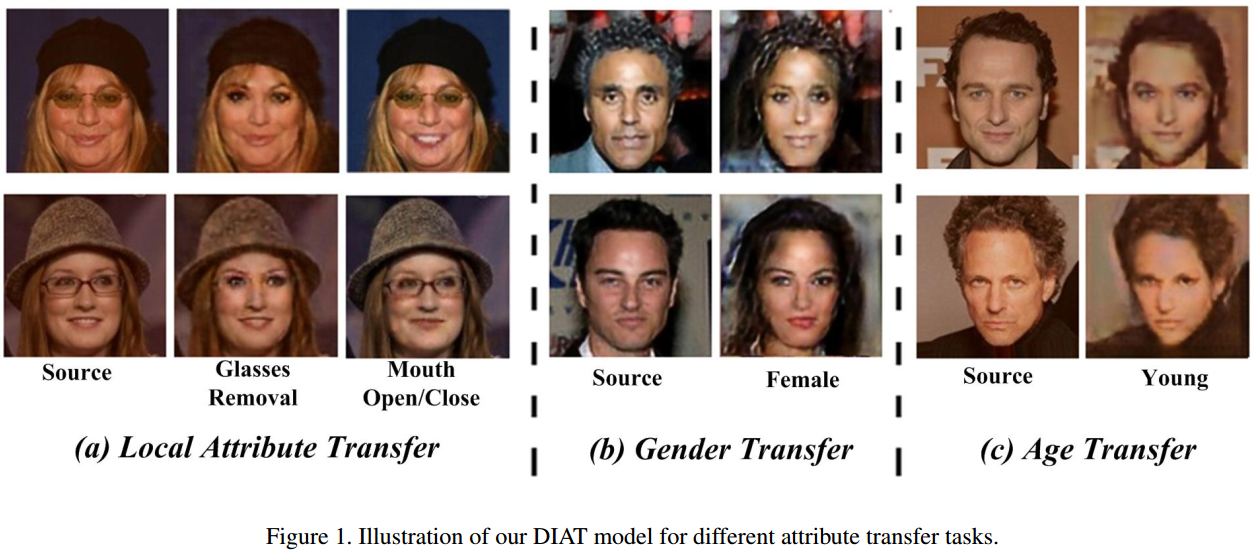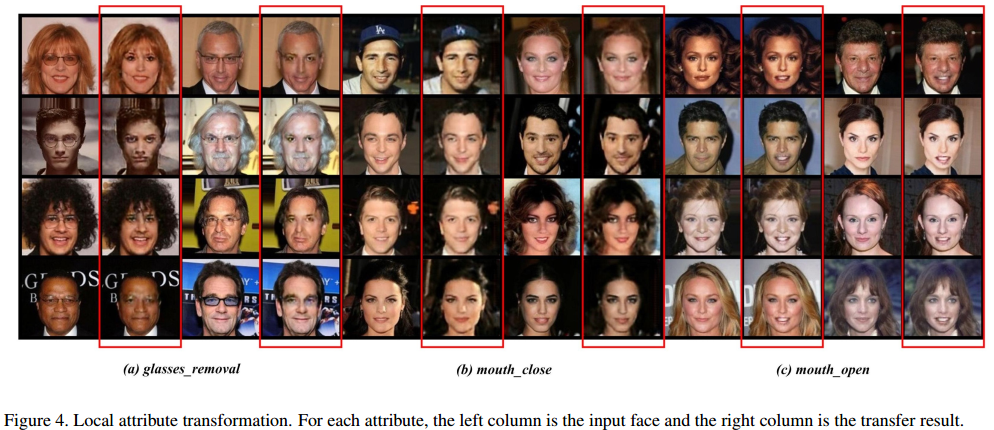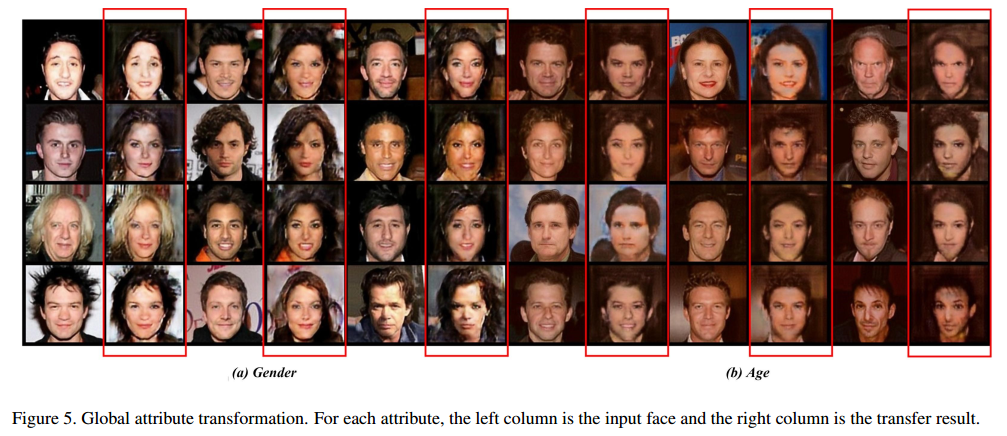## 4 Unsupervised Cross-Domain Image Generation(ICLR 2017 )

Recent achievements replicate some of these capabilities to some degree: Generative Adversarial Networks (GANs) are able to convincingly generate novel samples that match that of a given training set; style transfer methods are able to alter the visual style of images; domain adaptation methods are able to generalize learned functions to new domains even without labeled samples in the target domain and transfer learning is now commonly used to import existing knowledge and to make learning much more efficient.

These capabilities, however, do not address the general analogy synthesis problem that we tackle in this work. Namely, given separated but otherwise unlabeled samples from domains $S$ and $T$ and a perceptual function $f$, learn a mapping $G : S \to T$ such that $f(x) ∼ f(G(x)$

As a main application challenge, we tackle the problem of emoji generation for a given facial image. Despite a growing interest in emoji and the hurdle of creating such personal emoji manually, no system has been proposed, to our knowledge, that can solve this problem. Our method is able to produce face emoji that are visually appealing and capture much more of the facial characteristics than the emoji created by well-trained human annotators who use the conventional tools.

### 4.2 Model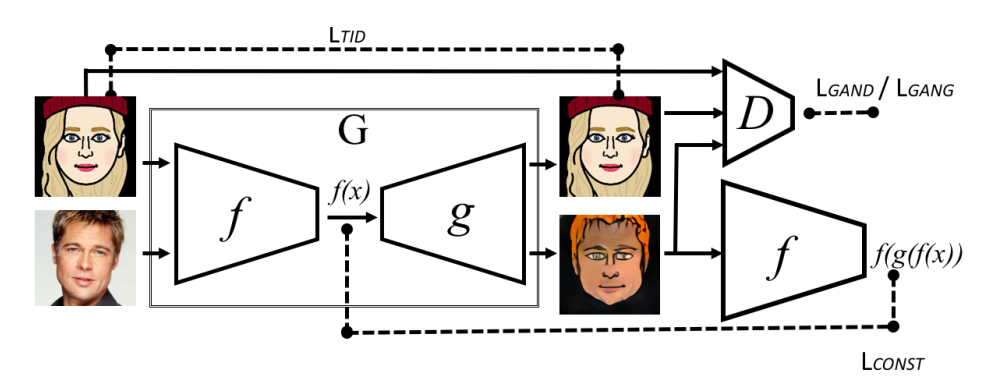Loss: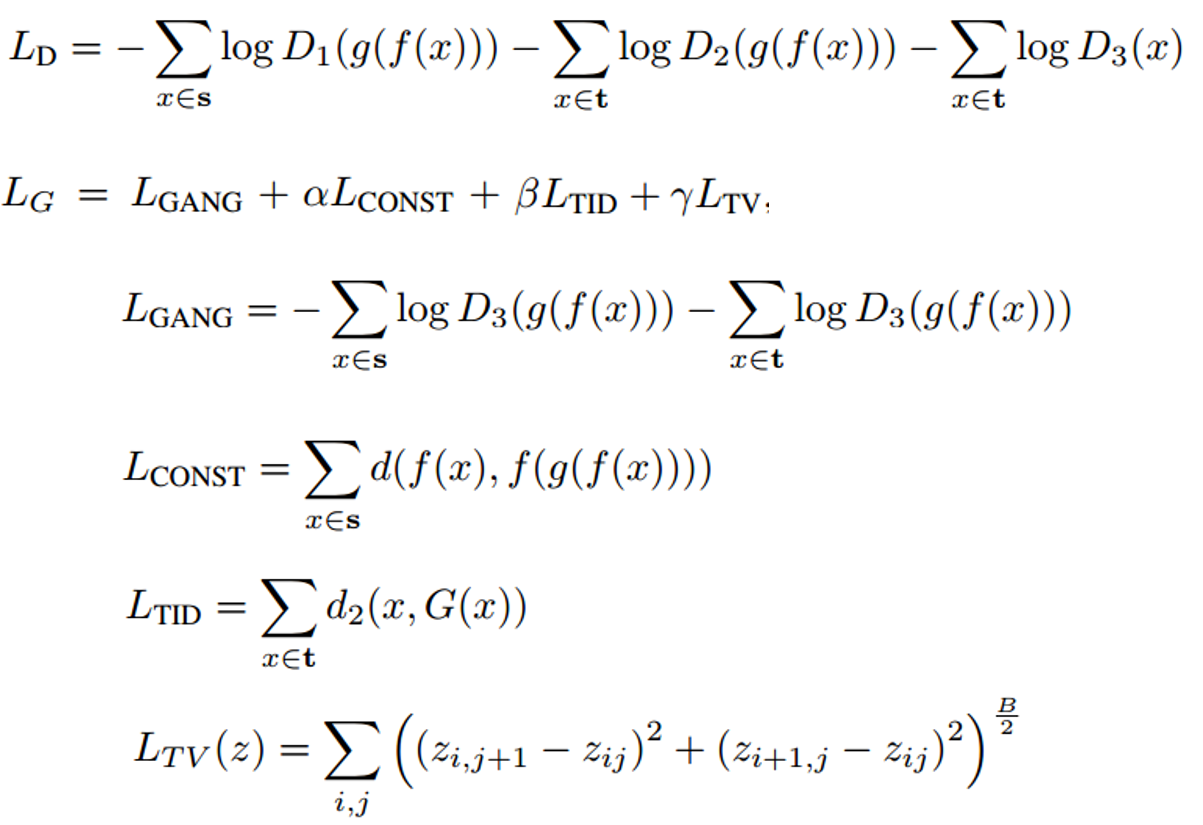1. $D$ is a ternary classification function from the domain $T$ to 1,2,3, and $D_i(x)$ is the
probability it assigns to class $i = 1,2,3$ for an input sample $x$
2. During optimization, $L_G$ is minimized over $g$ and $L_D$ is minimized over $D$
3. $L_{CONST}$ enforces f-constancy for $x \in S$, while $L_{TID}$ enforces that for samples $x \in T$
4. $L_{TV}$ is an anisotropic total variation loss, which is added in order to slightly smooth the resulting image
5. $f$ is trained use other datasets before training this model

### 4.3 Experiments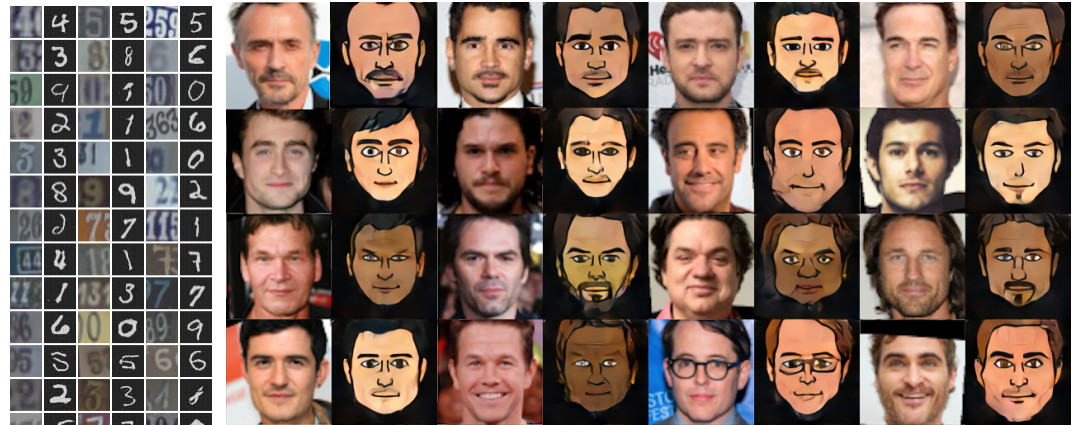Dataset:

1. Street View House Number (SVHN) dataset to the domain of the MNIST dataset
2. FROM PHOTOS TO EMOJI

Metrics: MNIST Accuracy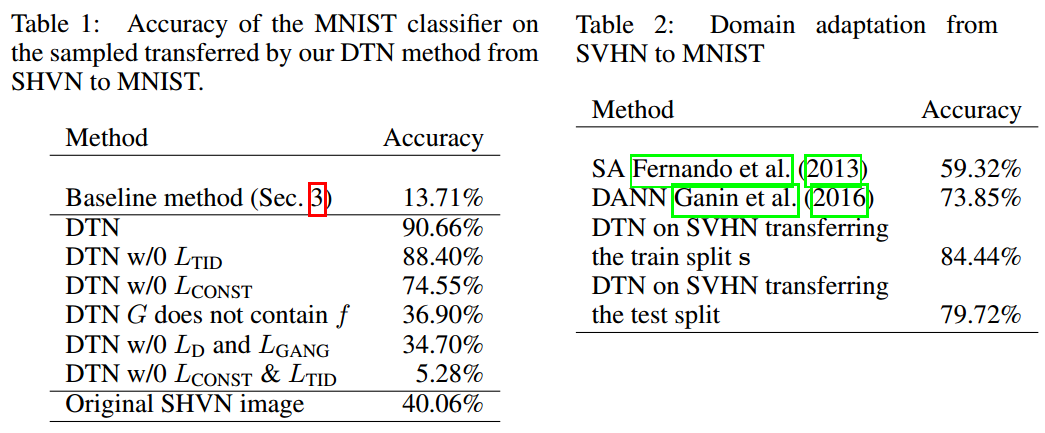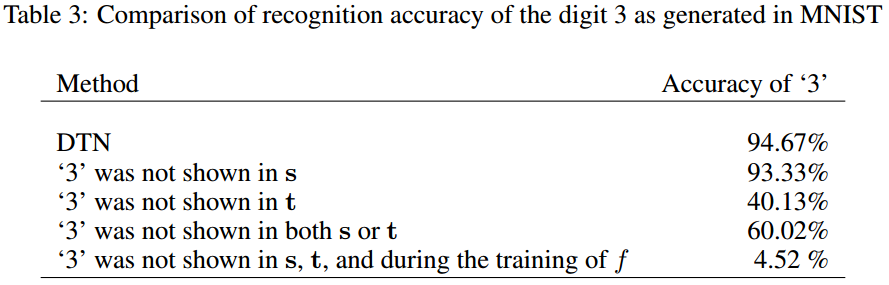## 5 StarGAN: Multi-Domain Image-to-Image Translation

### 5.1 Introduction

Recent studies have shown remarkable success in image-to-image translation for two domains. However, existing approaches have limited scalability and robustness in handling more than two domains, since different models should be built independently for every pair of image domains.

To address this limitation, we propose StarGAN, a novel and scalable approach that can perform image-to-image translations for multiple domains using only a single model

We can further extend to training multiple domains from different datasets.

### 5.2 Model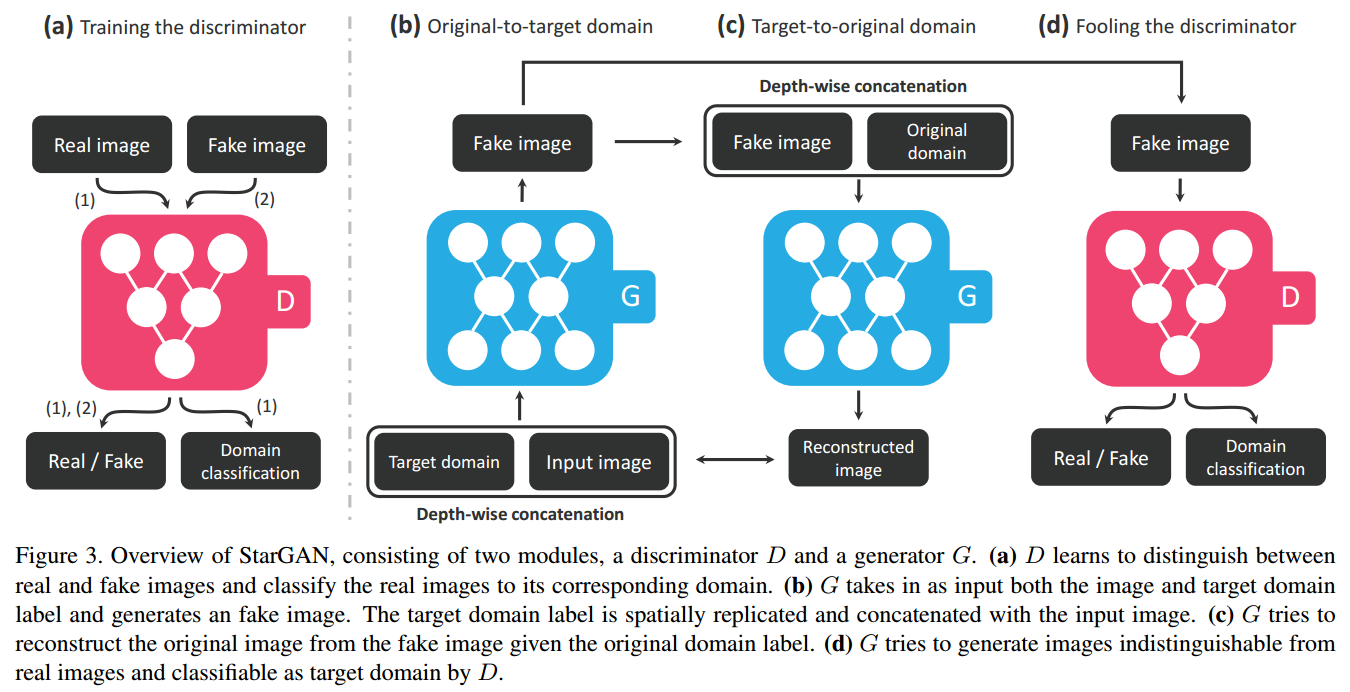Loss: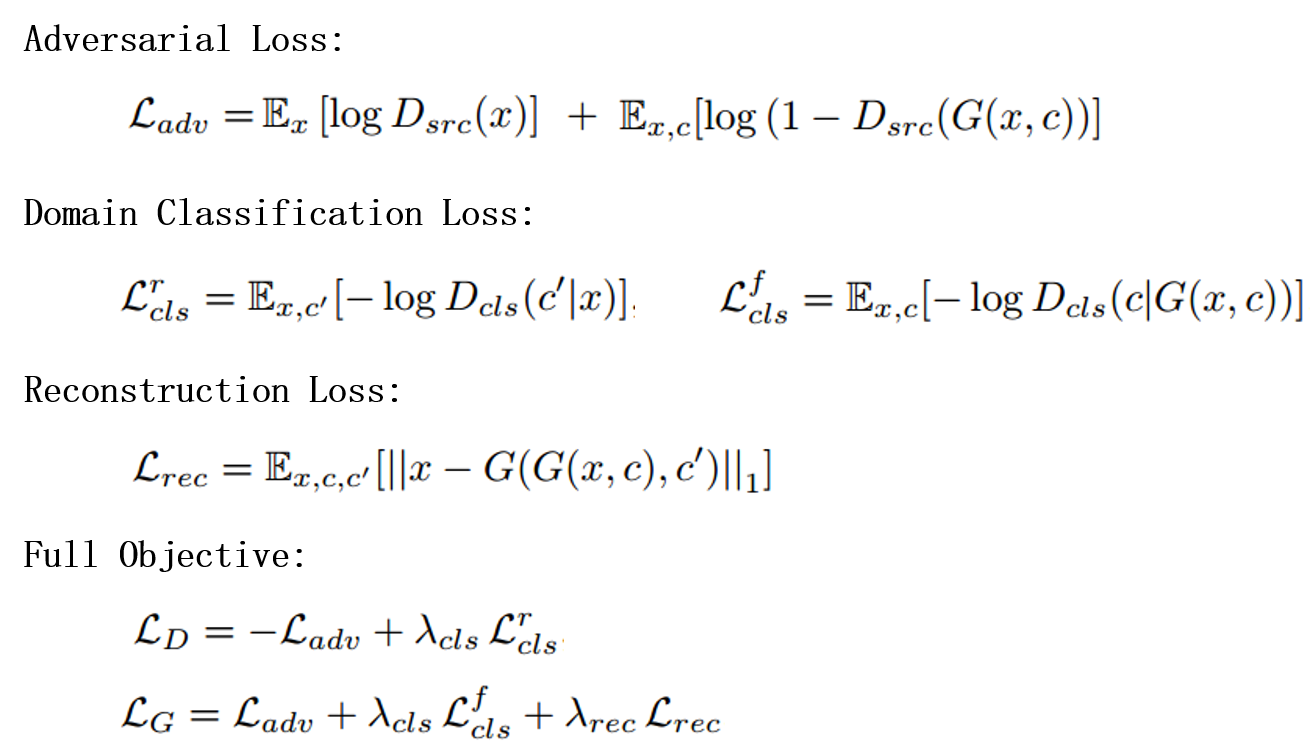1. a domain classification loss of real images($L_{cls}^r$) used to optimize D, and a domain classification loss of fake images($L_{cls}^f$) used to optimize G
2. Use $L_{rec}$ to guarantee that translated images preserve the content of its input images while changing only the domain-related part of the inputs.

### 5.3 Training with Multiple Datasets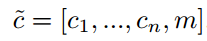In StarGAN, we use an n-dimensional one-hot vector to represent m, with n being the number of datasets. and $c_i$ represents a vector for the labels of the $i$-th dataset. The vector of the known label $c_i$ can be represented as either a binary vector for binary attributes or a one-hot vector for categorical attributes

#### 5.3.2 Training Strategy

When training StarGAN with multiple datasets, we use the domain label $\overset{\sim}{c}$ defined at above as input to the generator. By doing so, the generator learns to ignore the unspecified labels, which are zero vectors, and
focus on the explicitly given label. The structure of the generator is exactly the same as in training with a single dataset, except for the dimension of the input label $\overset{\sim}{c}$.

### 5.3.3 CelebA and RaFD dataset demo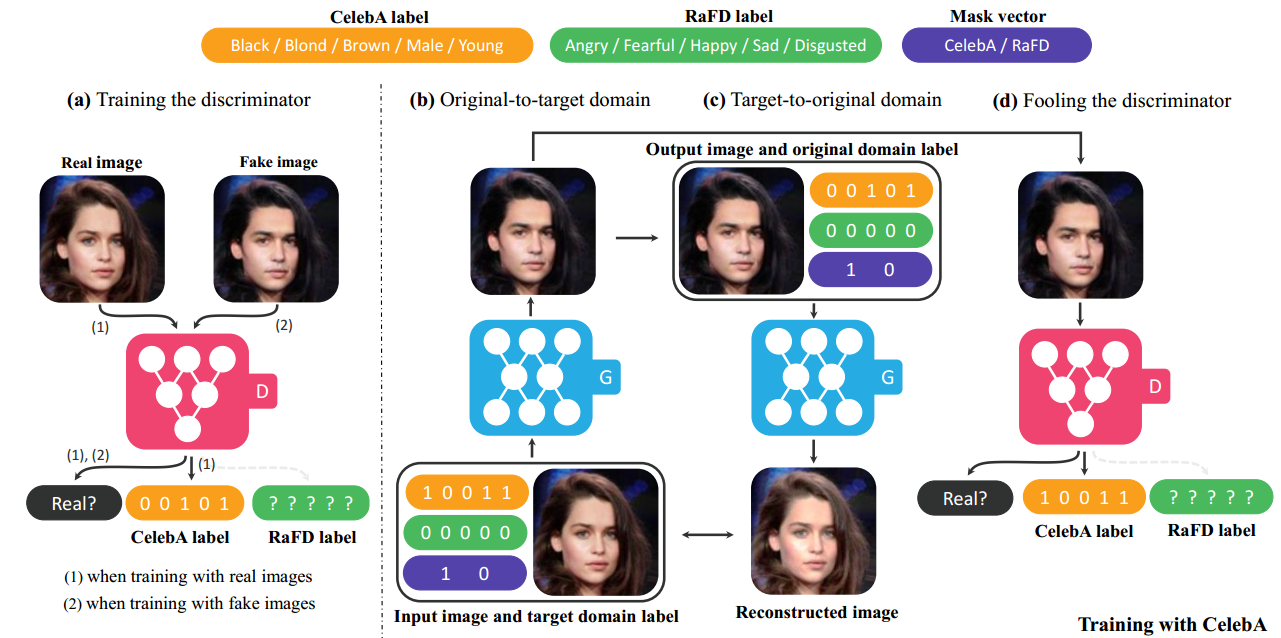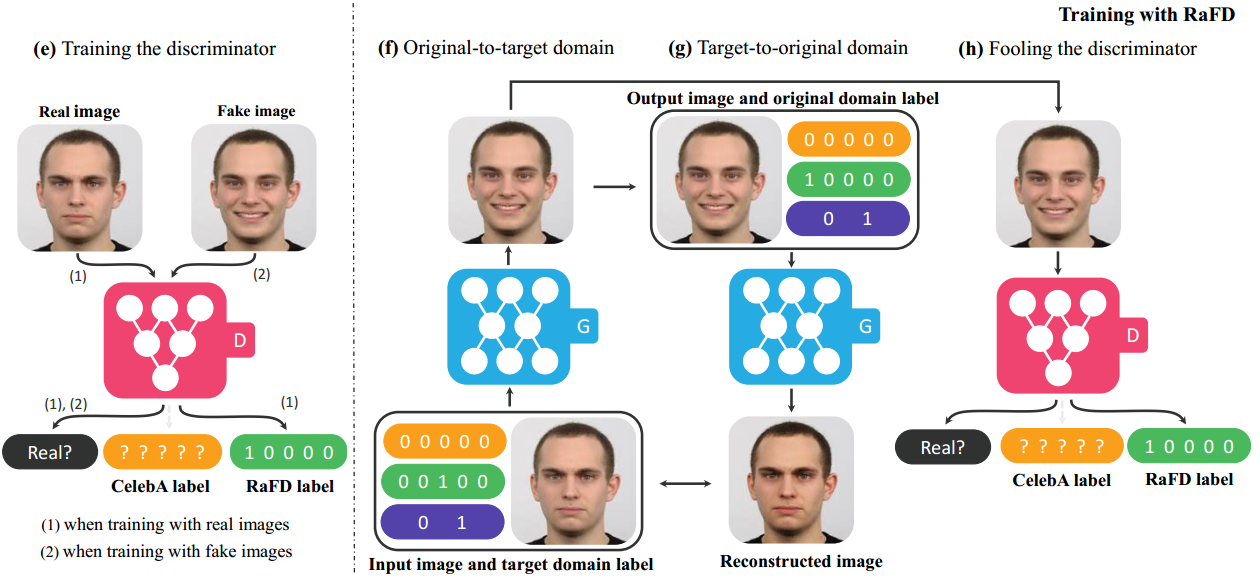### 5.4 Experiments

Dataset: CelebA, RaFD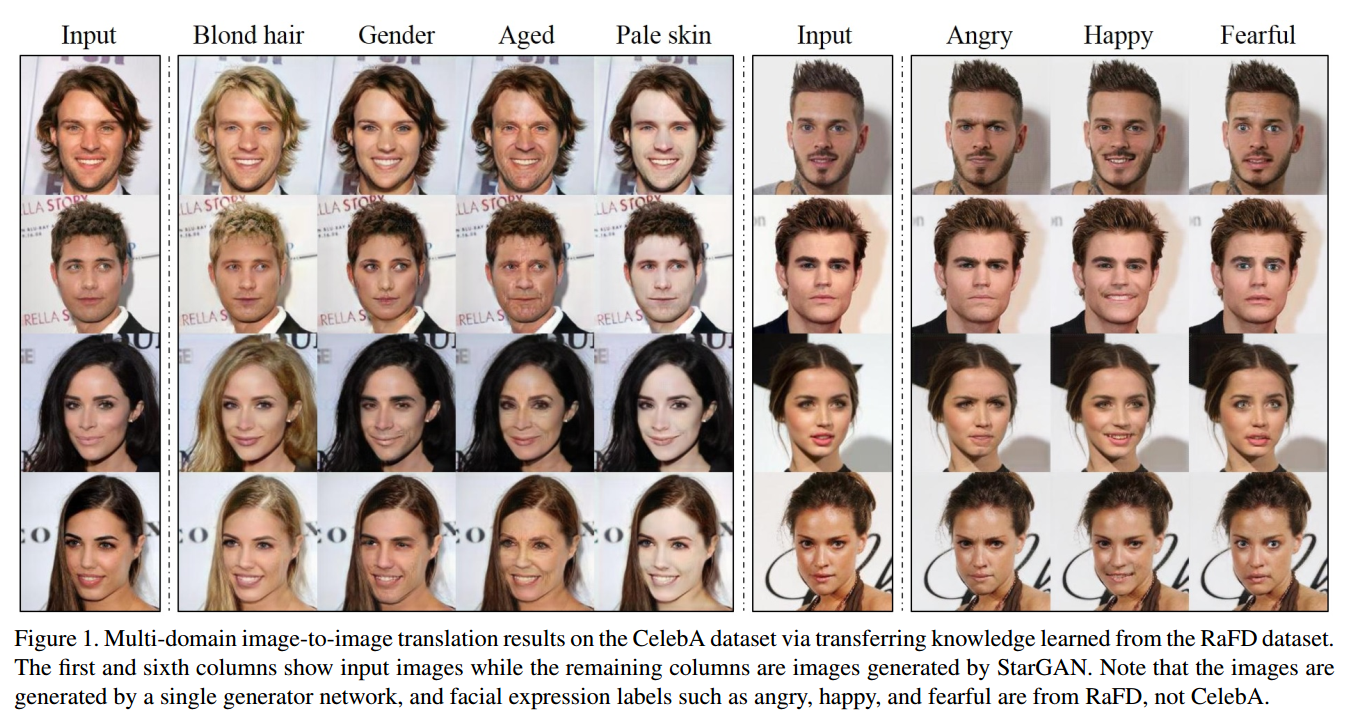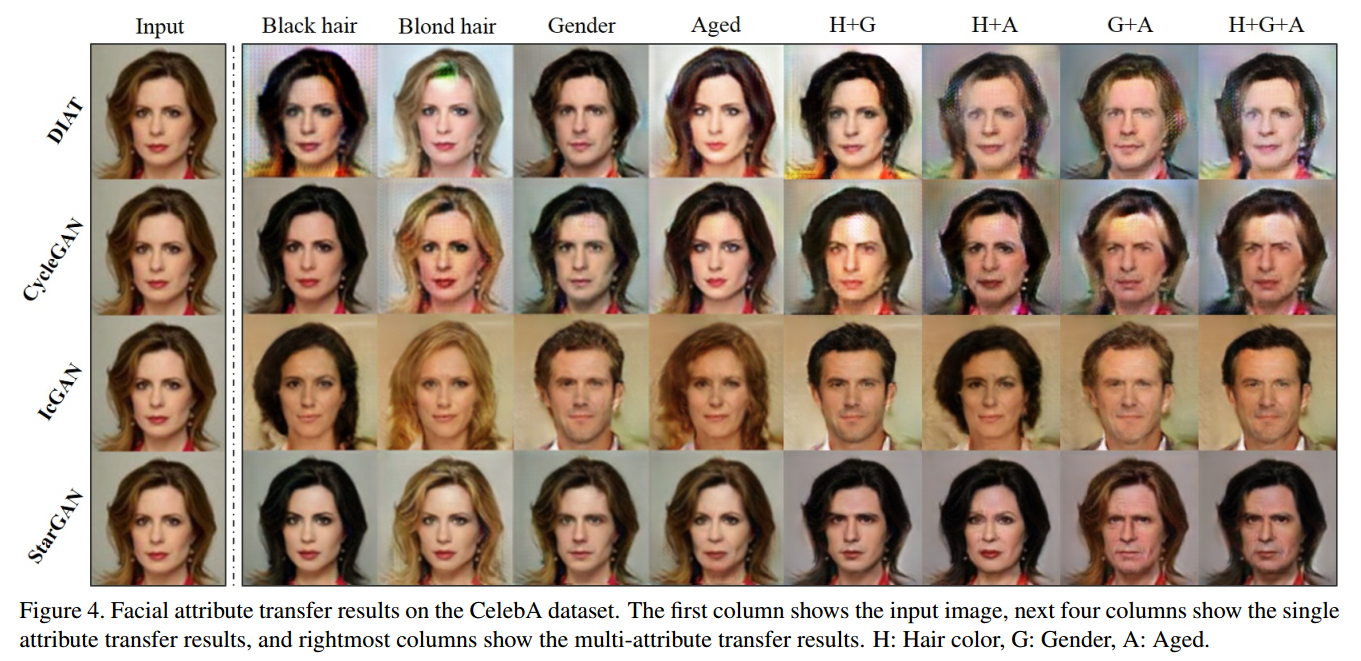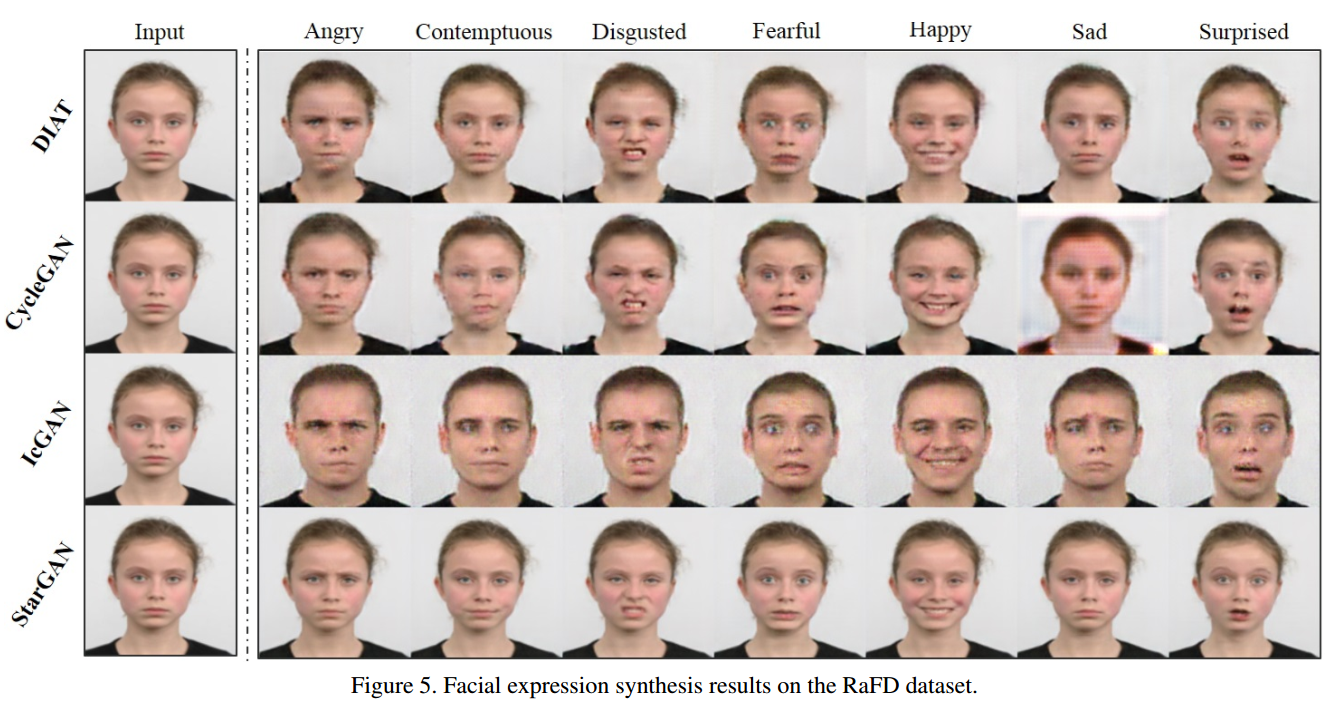Metrics: AMT(human evaluation)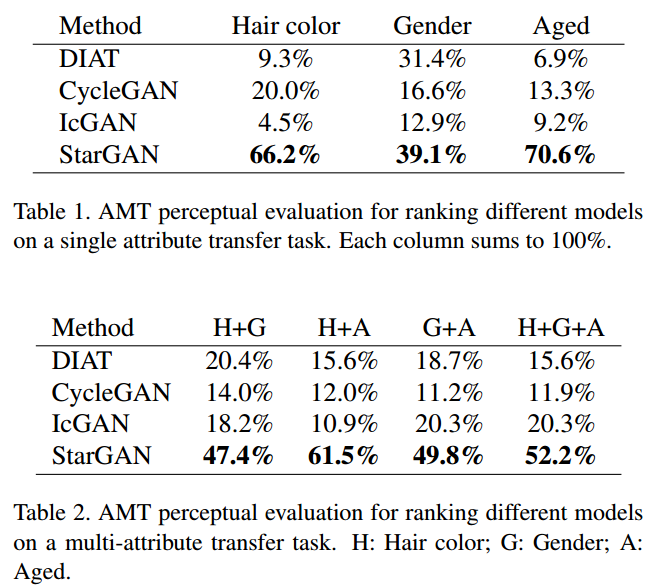Dataset: RaFD dataset (90%/10% splitting for training and test sets)

Metrics: compute the classification error of a facial expression on synthesized images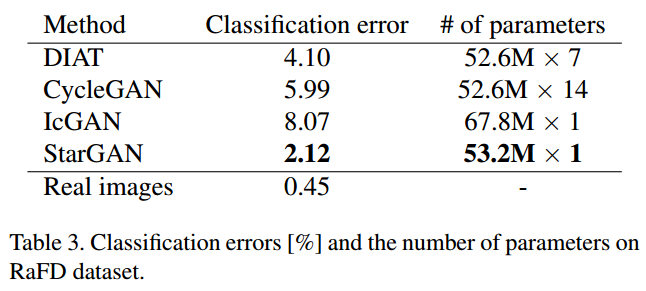## 6 Pix2Pix: Image-to-Image Translation with Conditional Adversarial Networks(use paired data)(CVPR 2017)

### 6.1 Introduction

We investigate conditional adversarial networks as a general-purpose solution to image-to-image translation problems. These networks not only learn the mapping from input image to output image, but also learn a loss function to train this mapping. This makes it possible to apply the same generic approach to problems that traditionally would require very different loss formulations.

we no longer hand-engineer our mapping functions, and this work suggests we can achieve reasonable results without hand-engineering our loss functions either

(One architecture to different works)

### 6.2 Model

#### 6.2.1 Generator with skips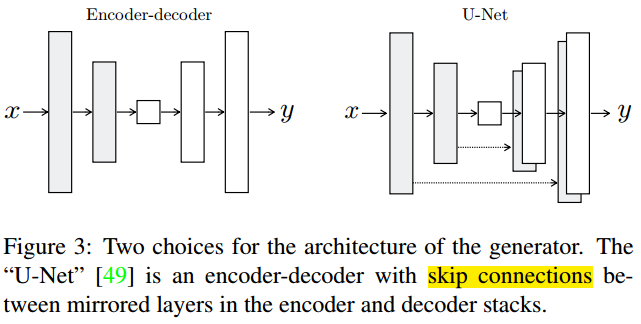#### 6.2.2 Conditional GANs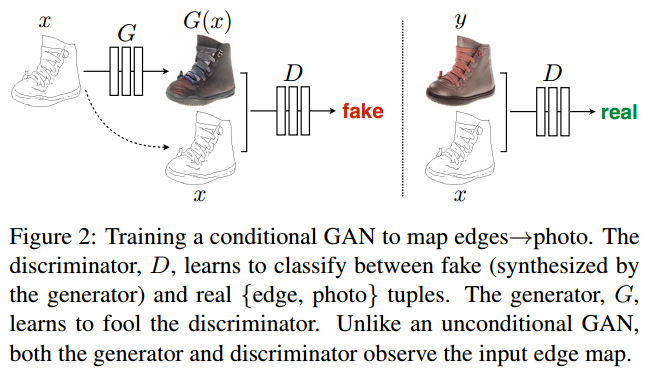#### 6.2.3 PatchGAN

It is well known that the L2 loss and L1produce blurry results on image generation problems . Although these losses fail to encourage high-frequency crispness, in many cases they nonetheless accurately capture the low frequencies .

In order to model high-frequencies, it is sufficient to restrict our attention to the structure in local image patches. Therefore, we design a discriminator architecture – which we term a PatchGAN – that only penalizes structure at the scale of patches. This discriminator tries to classify if each N × N patch in an image is real or fake. We run this discriminator convolutionally across the image, averaging all responses to provide the ultimate output of D

### 6.2.4 Loss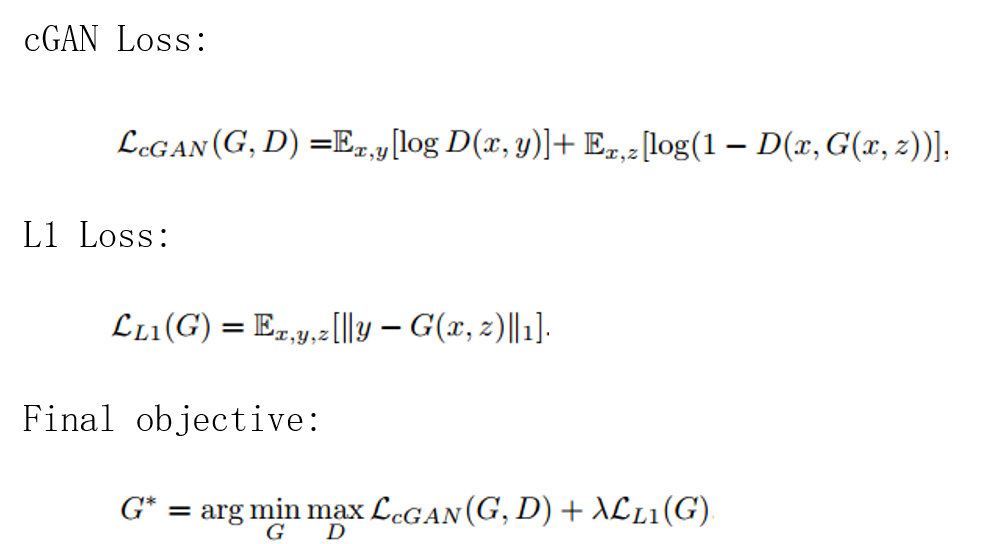Dataset:

1. Semantic labels$photo, trained on the Cityscapes dataset. 2. Architectural labels!photo, trained on CMP Facades 3. Map to aerial photo, trained on data scraped from Google Maps. 4. BW to color photos, trained on [50 Imagenet large scale visual recognition challenge]. 5. Edges to photo, trained on data from [64 Generative visual manipulation on the natural image manifold] and [59 Fine-Grained Visual Comparisons with Local Learning ]; binary edges generated using the HED edge detector [57 Holistically-nested edge detection ] plus post processing. 6. Sketch to photo: tests edges to photo models on human drawn sketches from [18 How do humans sketch objects]. 7. Day to night, trained on [32 Transient attributes for high-level understanding and editing of outdoor scenes ]. 8. Thermal to color photos, trained on data from [26 Multispectral pedestrian detection: Benchmark dataset and baseline]. 9. Photo with missing pixels to inpainted photo, trained on Paris StreetView from [13 What makes paris look like paris] Metrics: AMT, FCN-scores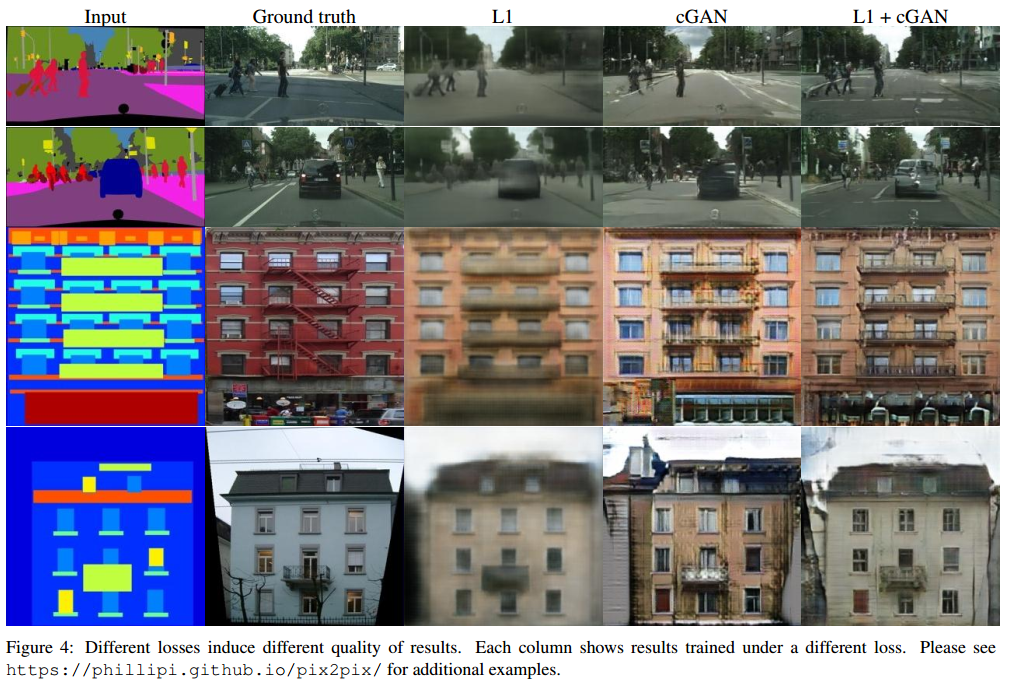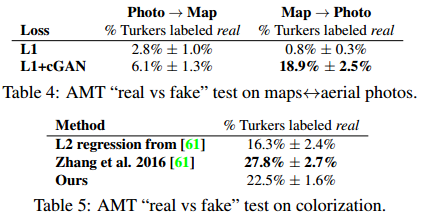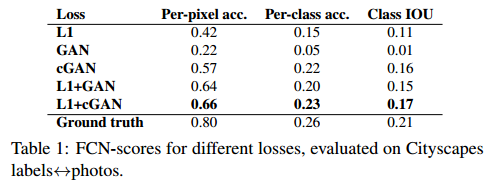## 7 Photo-Realistic Single Image Super-Resolution Using a GAN(use paired data to train)(CVPR 2017) ### 7.1 Task Despite the breakthroughs in accuracy and speed of single image super-resolution using faster and deeper convolutional neural networks, one central problem remains largely unsolved: how do we recover the finer texture details when we super-resolve at large upscaling factors? Recent work has largely focused on minimizing the mean squared reconstruction error. The resulting estimates have high peak signal-to-noise ratios, but they are often lacking high-frequency details and are perceptually unsatisfying in the sense that they fail to match the fidelity expected at the higher resolution. To our knowledge, it is the first framework capable of inferring photo-realistic natural images for 4× upscaling factors. To achieve this, we propose a perceptual loss function which consists of an adversarial loss and a content loss ### 7.2 Model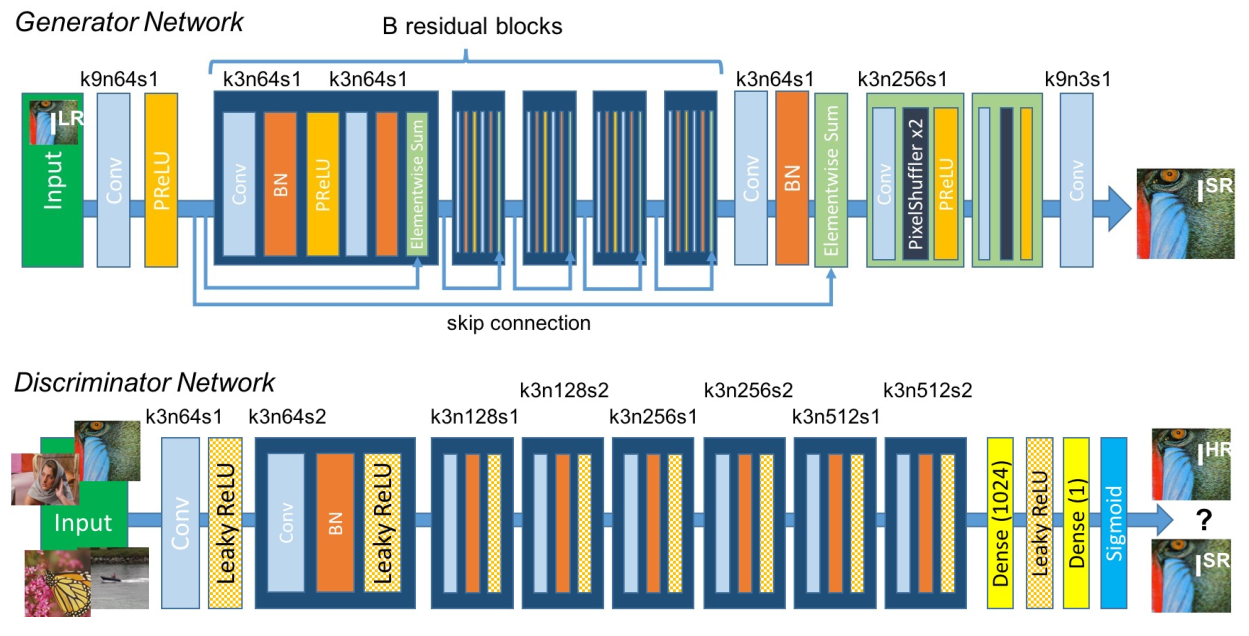Loss: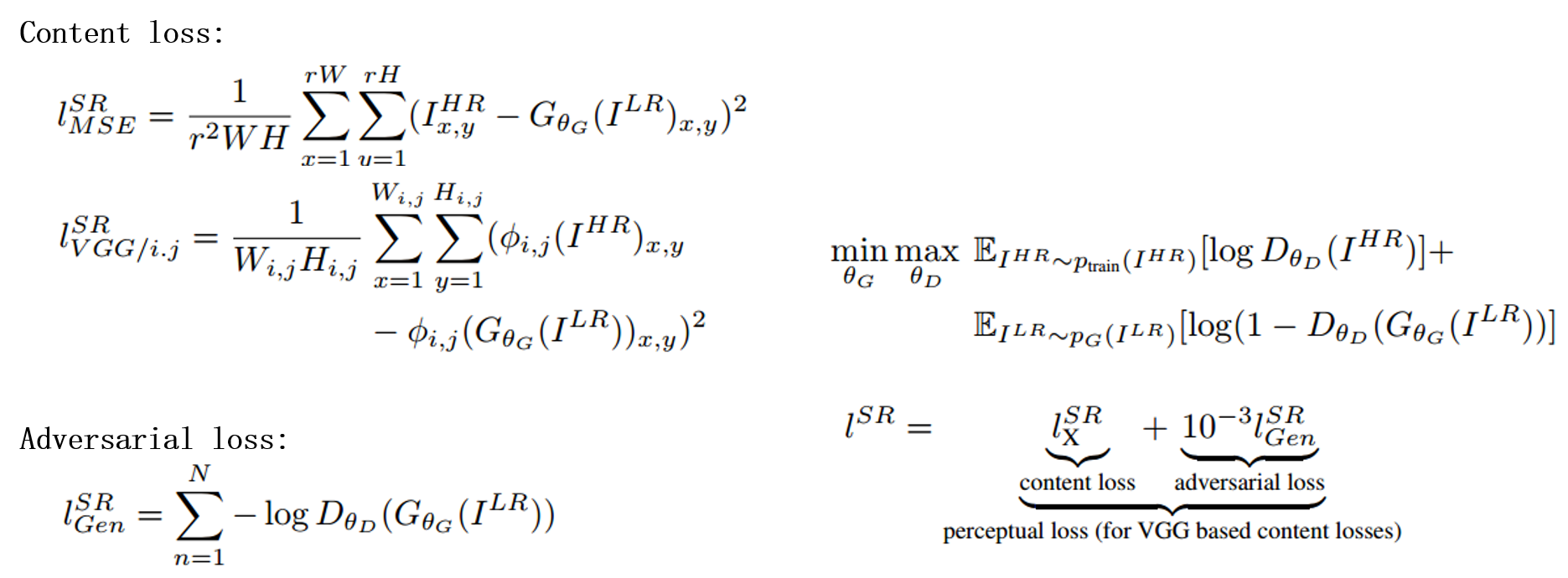1. {i,j}$ in $l{VGG/i,j}^{SR}$ , we indicate the feature map obtained by the j-th convolution (after activation) before the i-th max pooling layer within the VGG19 network
2. D network is optimized by the min-max game
3. G network is optimized by the loss $l^{SR}$

### 7.3 Experiments

Dataset:

1. Set5 [Low-complexity single-image super-resolution based on nonnegative neighbor embedding ],
2. Set14 [On single image scale-up using sparse-representations ]
3. BSD100
4. the testing set of BSD300

Metrics: Mean opinion score (MOS) testing(human evaluation)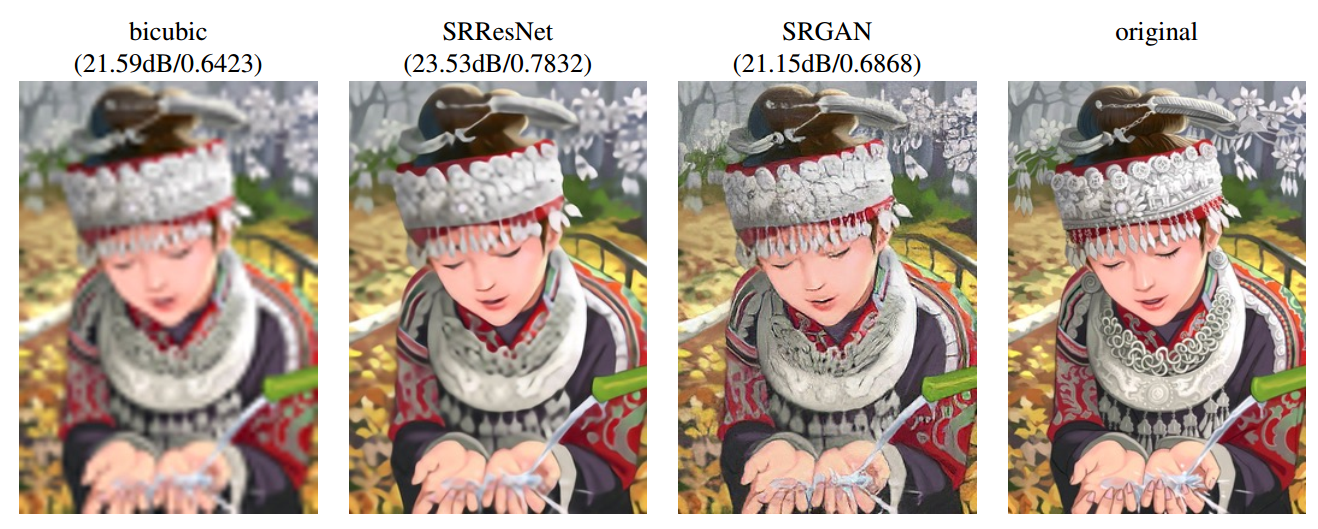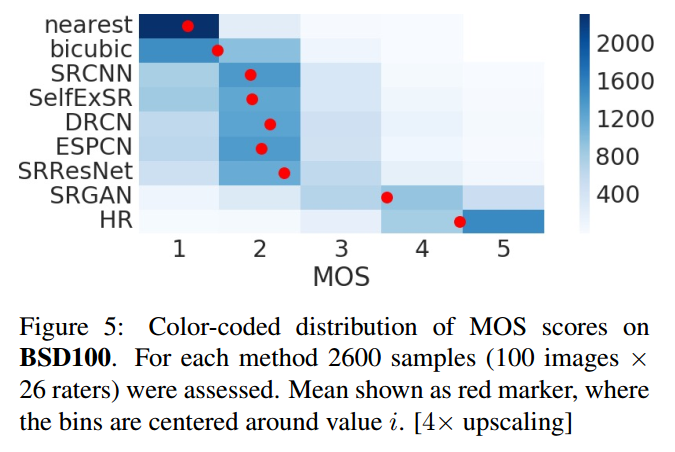## 8 Conclusion

### 8.1 Reason for using GAN

1. How to design effective loss
2. How to use unpaired data

1. No need of the specific loss, but a high level goal
2. Able to handle unpaired data

1. The Generator network often produce insensitive results
2. Mode collapse: all inputs are mapped to the same output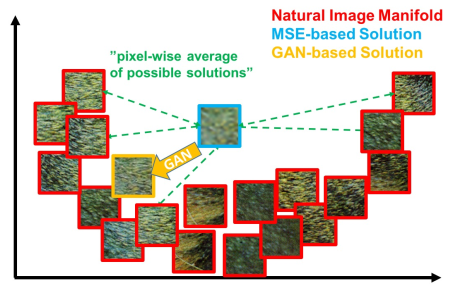### 8.2 Good ideas

• GAN Loss: keep high level domain feature
• Keep specific entity feature
• Given separated but otherwise unlabeled samples from domains $S$ and $T$ and a perceptual function $f$, learn a mapping $G : S \to T$ such that $f(x) ∼ f(G(x))$
1. Perceptual Loss
2. pre-trained f
• Cycle consistency
• Enhancement network
• Translations for multiple domains using only a single model

### 8.3 Metrics

• Human evaluation: AMT, MOS
• Visualizing the generated results
• Use a model in the target domain to evaluate: FCN Scores(MNIST classifiers, VGG face classifier)